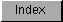```
There are six math libraries providing functions ranging from adding two
floating point numbers to calculating a hyperbolic cosine.  They are:

mathffp.library
the basic function library

mathtrans.library
the FFP transcendental math library

mathieeesingbas.library
the IEEE single-precision library

mathieesingtrans.library
the IEEE single-precision transcendental library

mathieeedoubbas.library
the IEEE double-precision library

mathieesingtrans.library
the IEEE double-precision transcendental library

```